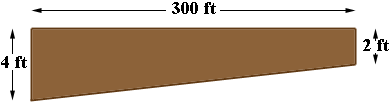SEARCH HOMEMath Central Quandaries & QueriesQuestion from John: I am trying to figure out how much flowfill I will need for a ditch that we are digging out. The ditch is 300' long and is 4' deep on one end and 2' deep on the other end. We are going to dig this ditch out and replace it with a flowfill concrete material. How much flowfill material will we need or what is going to be the volume of this ditch?John,

How wide is the ditch? Are the sides straight up and down?

Penny

The ditch is 2’ wide and yes the sides are straight up and down. Thank you, John

John,

The volume of the ditch is the area of the cross-section times the width of 2 feet.If the slope from the deep end to the shallow end is gradual then the cross-section is a trapezoid.The area of a trapezoid is the average of the lengths of the parallel sides times the distance between the parallel sides. This in your situation is

(4 + 2)/2 × 300 = 900 square feet.

Thus the volume of the ditch is

900 × 2 = 1800 cubic feet.

There are 27 cubic feet in a cubic yard so the volume is 1800/27 = 66.67 cubic yards.

PennyMath Central is supported by the University of Regina and The Pacific Institute for the Mathematical Sciences.